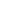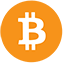BTC: \$ 27568.98 (0.98%)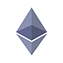ETH: \$ 1641.15 (-0.50%)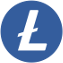LTC: \$ 63.98 (-2.33%)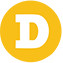DOGE: \$ 0.06102 (-0.45%)

We will soon release our new game Flash Roulette! To make this game provably fair, we announce our methods here. A chain of 1 million SHA512 hashes was generated (starting with a server secret.) The final hash is: 4c28a546da313d86a9ca42c59e833c179275ae0397893e92685ff414a17fe1ee963623a18c673171e0ee77204252c8fa0b7f629630861f53ea7b94a13f205e9b Bitsler will go through the hashes (in reverse order) and use each hash to determine the roulette result. So the hash of our first game's hash is the same as our published final hash. To ensure we didn't pick a hash chain that has some advantage for us, we will use a future bitcoin block hash as "client seed" to generate the final roulette results. We will use the block hash of bitcoin block https://btc.com/block/668720 (around 8 AM GMT at Feb 2, 2020.) The code to generate each roulette result is:
``````\$hash = hash_hmac('sha512', \$gameHash, \$blockHash);
\$config_multipliers =
[
[
"150" => 3,
"100" => 8,
"50" => 5
],
[
"350" => 1,
"250" => 1,
"200" => 1,
"150" => 1,
"100" => 2,
"50" => 2,
"29" => 8
],
[
"350" => 1,
"250" => 1,
"200" => 1,
"150" => 1,
"100" => 2,
"50" => 2,
"29" => 8
],
[
"350" => 1,
"250" => 1,
"200" => 1,
"150" => 1,
"100" => 2,
"50" => 2,
"29" => 8
]
];    \$offset = 0;
do {
\$number = substr(\$hash, \$offset, 5);
\$number = hexdec(\$number);
\$offset += 5;
} while (\$number > 999999);
\$number = \$number % 37;    \$multipliers = [];
for (\$i = 0; \$i < count(\$config_multipliers); \$i++) {
do {
\$multiNumber = substr(\$hash, \$offset, 5);
\$multiNumber = hexdec((\$multiNumber));
\$offset += 5;
} while (\$multiNumber > 999999 || isset(\$multipliers[\$multiNumber % 37]));
\$multiNumber = \$multiNumber % 37;
// multiplier
\$amount_number = hexdec(substr(\$hash, \$offset, 1));
\$offset += 1;
// Loop multipliers
\$multiplier_number = 0;
foreach (\$config_multipliers[\$i] as \$multiplier => \$range) {
\$multiplier_number += \$range;
// If the end of range is more than generated number, we use current multiplier
if (\$multiplier_number > \$amount_number) {
\$multiplier = floatval(\$multiplier);
break;
}
}        // return
\$multipliers[\$multiNumber] = \$multiplier;
}
print_r(["result" => \$number, "multipliers" => \$multipliers]);``````

## !Important

This blog post will be shared on our social media channels and archived by several archive websites. Therefor we cannot edit the final hash (and therefor hash chain) nor the future bitcoin block number anymore. This makes our new game Flash Roulette completely provably fair.